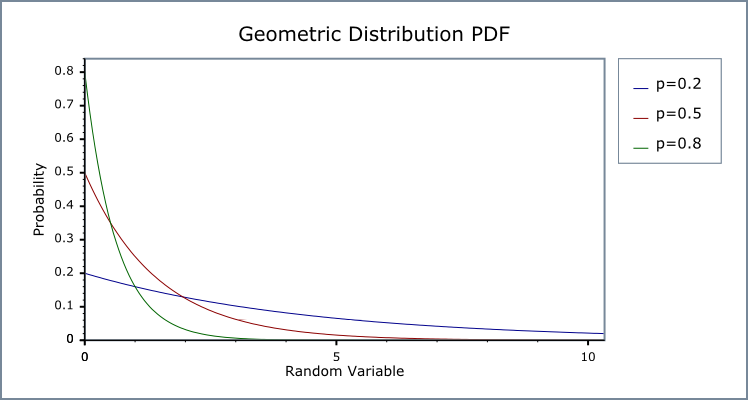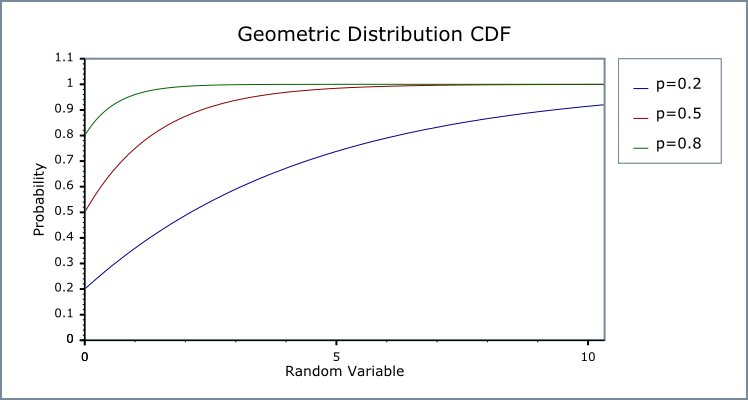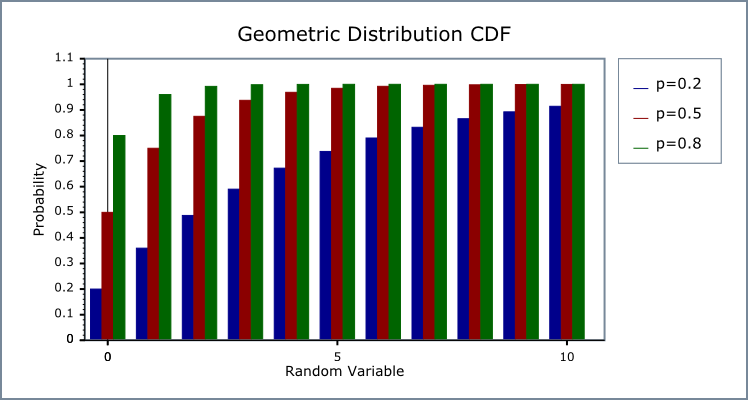Boost C++ Libraries

...one of the most highly regarded and expertly designed C++ library projects in the world.

This is the documentation for an old version of boost. Click here for the latest Boost documentation.
Geometric Distribution

#include <boost/math/distributions/geometric.hpp>

namespace boost{ namespace math{

template <class RealType = double,
class Policy   = policies::policy<> >
class geometric_distribution;

typedef geometric_distribution<> geometric;

template <class RealType, class Policy>
class geometric_distribution
{
public:
typedef RealType value_type;
typedef Policy   policy_type;
// Constructor from success_fraction:
geometric_distribution(RealType p);

// Parameter accessors:
RealType success_fraction() const;
RealType successes() const;

// Bounds on success fraction:
static RealType find_lower_bound_on_p(
RealType trials,
RealType successes,
RealType probability); // alpha
static RealType find_upper_bound_on_p(
RealType trials,
RealType successes,
RealType probability); // alpha

// Estimate min/max number of trials:
static RealType find_minimum_number_of_trials(
RealType k,     // Number of failures.
RealType p,     // Success fraction.
RealType probability); // Probability threshold alpha.
static RealType find_maximum_number_of_trials(
RealType k,     // Number of failures.
RealType p,     // Success fraction.
RealType probability); // Probability threshold alpha.
};

}} // namespaces

The class type geometric_distribution represents a geometric distribution: it is used when there are exactly two mutually exclusive outcomes of a Bernoulli trial: these outcomes are labelled "success" and "failure".

For Bernoulli trials each with success fraction p, the geometric distribution gives the probability of observing k trials (failures, events, occurrences, or arrivals) before the first success.

NoteFor this implementation, the set of trials includes zero (unlike another definition where the set of trials starts at one, sometimes named shifted).

The geometric distribution assumes that success_fraction p is fixed for all k trials.

The probability that there are k failures before the first success is

Pr(Y=k) = (1-p)kp

For example, when throwing a 6-face dice the success probability p = 1/6 = 0.1666 ̇  . Throwing repeatedly until a three appears, the probability distribution of the number of times not-a-three is thrown is geometric.

Geometric distribution has the Probability Density Function PDF:

(1-p)kp

The following graph illustrates how the PDF and CDF vary for three examples of the success fraction p, (when considering the geometric distribution as a continuous function),and as discrete.Related Distributions

The geometric distribution is a special case of the Negative Binomial Distribution with successes parameter r = 1, so only one first and only success is required : thus by definition    geometric(p) == negative_binomial(1, p)

negative_binomial_distribution(RealType r, RealType success_fraction);
negative_binomial nb(1, success_fraction);
geometric g(success_fraction);
ASSERT(pdf(nb, 1) == pdf(g, 1));

This implementation uses real numbers for the computation throughout (because it uses the real-valued power and exponential functions). So to obtain a conventional strictly-discrete geometric distribution you must ensure that an integer value is provided for the number of trials (random variable) k, and take integer values (floor or ceil functions) from functions that return a number of successes.

CautionThe geometric distribution is a discrete distribution: internally, functions like the cdf and pdf are treated "as if" they are continuous functions, but in reality the results returned from these functions only have meaning if an integer value is provided for the random variate argument. The quantile function will by default return an integer result that has been rounded outwards. That is to say lower quantiles (where the probability is less than 0.5) are rounded downward, and upper quantiles (where the probability is greater than 0.5) are rounded upwards. This behaviour ensures that if an X% quantile is requested, then at least the requested coverage will be present in the central region, and no more than the requested coverage will be present in the tails. This behaviour can be changed so that the quantile functions are rounded differently, or even return a real-valued result using Policies. It is strongly recommended that you read the tutorial Understanding Quantiles of Discrete Distributions before using the quantile function on the geometric distribution. The reference docs describe how to change the rounding policy for these distributions.
Member Functions
Constructor
geometric_distribution(RealType p);

Constructor: p or success_fraction is the probability of success of a single trial.

Requires: 0 <= p <= 1.

Accessors
RealType success_fraction() const; // successes / trials (0 <= p <= 1)

Returns the success_fraction parameter p from which this distribution was constructed.

RealType successes() const; // required successes always one,
// included for compatibility with negative binomial distribution
// with successes r == 1.

Returns unity.

The following functions are equivalent to those provided for the negative binomial, with successes = 1, but are provided here for completeness.

The best method of calculation for the following functions is disputed: see Binomial Distribution and Negative Binomial Distribution for more discussion.

Lower Bound on success_fraction Parameter p
static RealType find_lower_bound_on_p(
RealType failures,
RealType probability) // (0 <= alpha <= 1), 0.05 equivalent to 95% confidence.

Returns a lower bound on the success fraction:

failures

The total number of failures before the 1st success.

alpha

The largest acceptable probability that the true value of the success fraction is less than the value returned.

For example, if you observe k failures from n trials the best estimate for the success fraction is simply 1/n, but if you want to be 95% sure that the true value is greater than some value, pmin, then:

pmin = geometric_distribution<RealType>::
find_lower_bound_on_p(failures, 0.05);

This function uses the Clopper-Pearson method of computing the lower bound on the success fraction, whilst many texts refer to this method as giving an "exact" result in practice it produces an interval that guarantees at least the coverage required, and may produce pessimistic estimates for some combinations of failures and successes. See:

Upper Bound on success_fraction Parameter p
static RealType find_upper_bound_on_p(
RealType trials,
RealType alpha); // (0 <= alpha <= 1), 0.05 equivalent to 95% confidence.

Returns an upper bound on the success fraction:

trials

The total number of trials conducted.

alpha

The largest acceptable probability that the true value of the success fraction is greater than the value returned.

For example, if you observe k successes from n trials the best estimate for the success fraction is simply k/n, but if you want to be 95% sure that the true value is less than some value, pmax, then:

pmax = geometric_distribution<RealType>::find_upper_bound_on_p(
k, 0.05);

This function uses the Clopper-Pearson method of computing the lower bound on the success fraction, whilst many texts refer to this method as giving an "exact" result in practice it produces an interval that guarantees at least the coverage required, and may produce pessimistic estimates for some combinations of failures and successes. See:

Estimating Number of Trials to Ensure at Least a Certain Number of Failures
static RealType find_minimum_number_of_trials(
RealType k,     // number of failures.
RealType p,     // success fraction.
RealType alpha); // probability threshold (0.05 equivalent to 95%).

This functions estimates the number of trials required to achieve a certain probability that more than k failures will be observed.

k

The target number of failures to be observed.

p

The probability of success for each trial.

alpha

The maximum acceptable risk that only k failures or fewer will be observed.

For example:

geometric_distribution<RealType>::find_minimum_number_of_trials(10, 0.5, 0.05);

Returns the smallest number of trials we must conduct to be 95% (1-0.05) sure of seeing 10 failures that occur with frequency one half.

This function uses numeric inversion of the geometric distribution to obtain the result: another interpretation of the result is that it finds the number of trials (failures) that will lead to an alpha probability of observing k failures or fewer.

Estimating Number of Trials to Ensure a Maximum Number of Failures or Less
static RealType find_maximum_number_of_trials(
RealType k,     // number of failures.
RealType p,     // success fraction.
RealType alpha); // probability threshold (0.05 equivalent to 95%).

This functions estimates the maximum number of trials we can conduct and achieve a certain probability that k failures or fewer will be observed.

k

The maximum number of failures to be observed.

p

The probability of success for each trial.

alpha

The maximum acceptable risk that more than k failures will be observed.

For example:

geometric_distribution<RealType>::find_maximum_number_of_trials(0, 1.0-1.0/1000000, 0.05);

Returns the largest number of trials we can conduct and still be 95% sure of seeing no failures that occur with frequency one in one million.

This function uses numeric inversion of the geometric distribution to obtain the result: another interpretation of the result, is that it finds the number of trials that will lead to an alpha probability of observing more than k failures.

Non-member Accessors

All the usual non-member accessor functions that are generic to all distributions are supported: Cumulative Distribution Function, Probability Density Function, Quantile, Hazard Function, Cumulative Hazard Function, mean, median, mode, variance, standard deviation, skewness, kurtosis, kurtosis_excess, range and support.

However it's worth taking a moment to define what these actually mean in the context of this distribution:

Table 14. Meaning of the non-member accessors.

Function

Meaning

The probability of obtaining exactly k failures from k trials with success fraction p. For example:

pdf(geometric(p), k)

The probability of obtaining k failures or fewer from k trials with success fraction p and success on the last trial. For example:

cdf(geometric(p), k)

The probability of obtaining more than k failures from k trials with success fraction p and success on the last trial. For example:

cdf(complement(geometric(p), k))

The greatest number of failures k expected to be observed from k trials with success fraction p, at probability P. Note that the value returned is a real-number, and not an integer. Depending on the use case you may want to take either the floor or ceiling of the real result. For example:

quantile(geometric(p), P)

The smallest number of failures k expected to be observed from k trials with success fraction p, at probability P. Note that the value returned is a real-number, and not an integer. Depending on the use case you may want to take either the floor or ceiling of the real result. For example:

quantile(complement(geometric(p), P))

Accuracy

This distribution is implemented using the pow and exp functions, so most results are accurate within a few epsilon for the RealType. For extreme values of double p, for example 0.9999999999, accuracy can fall significantly, for example to 10 decimal digits (from 16).

Implementation

In the following table, p is the probability that any one trial will be successful (the success fraction), k is the number of failures, p is the probability and q = 1-p, x is the given probability to estimate the expected number of failures using the quantile.

Function

Implementation Notes

pdf

pdf = p * pow(q, k)

cdf

cdf = 1 - qk=1

cdf complement

exp(log1p(-p) * (k+1))

quantile

k = log1p(-x) / log1p(-p) -1

quantile from the complement

k = log(x) / log1p(-p) -1

mean

(1-p)/p

variance

(1-p)/p²

mode

0

skewness

(2-p)/√q

kurtosis

9+p²/q

kurtosis excess

6 +p²/q

parameter estimation member functions

find_lower_bound_on_p

find_upper_bound_on_p

find_minimum_number_of_trials

find_maximum_number_of_trials